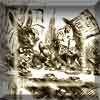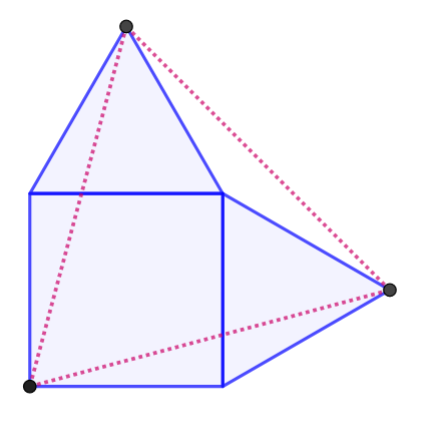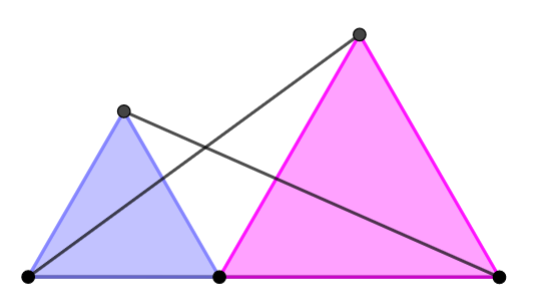#### You may also like### A Problem of Time

Consider a watch face which has identical hands and identical marks for the hours. It is opposite to a mirror. When is the time as read direct and in the mirror exactly the same between 6 and 7?The points P, Q, R and S are the midpoints of the edges of a non-convex quadrilateral.What do you notice about the quadrilateral PQRS and its area?

# Same Length

##### Age 11 to 16Challenge Level
For each problem, draw yourself a nice clear diagram.Here are some useful questions to ask yourself when working on geometry proofs:
• What angles do you know? (Mark them on your diagram.)
• Which lengths are equal? (Mark them on your diagram.)
• Which unknown angles are equal? How do you know?
• Are there any congruent triangles?